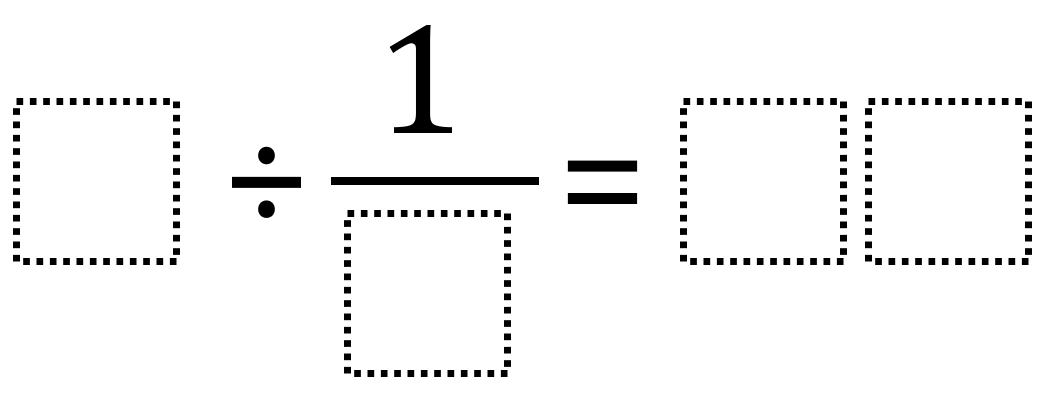# Dividing Fractions 4

Directions: Using the digits 1 through 9, at most one time each, place a digit in each box to create an equation with the greatest possible quotient.### Hint

How can dividing by a single digit by a fraction result in a two-digit quotient?

The greatest possible quotient is 72 from either 9 ÷ 1/8 = 72 or 8 ÷ 1/9 = 72.

Source: Robert Kaplinsky

## Equivalent Expressions with Fractions

Directions: Using the digits 0 to 9 at most one time each and choosing either …

1.ndyskndufkndsnjfoisonfslkjfsojndkndjndlkjdlkndnfnfnf

2.3.What is fractions?

4.What is fractions? I really dont get it

5.same this makes no sense

6.Cool problem

7.Oh now i get fractions, sorry im used to teaching kindergarten!

8.this is easy, 72. tooooo easy

•Nice job